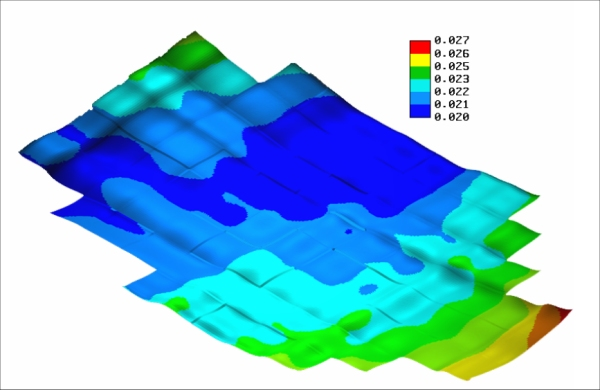### Quality Control of DFHRS Database Computation

In both cases - the geometrical approach implemented in the DFHRS software version 4.2, as well as the integrated geometricalphysical approach implemented in the DFHRS software version 5.0 - the functional models (2a-f) and ((4d)-(6)) respectively, mean and over-determined system of equations with respect to the parameters, and require parameter-estimations for redundant equation systems. The DFHRS software version 4.3 and version 5.0 solve the respective parameter estimation by a least squares adjustment (also called L2-norm estimation). The L2-norm is optimal - in the sense of a Maximum-Likelihood parameter estimation (M-estimation)  in the case of assumed multivariate normal distributed errors v. Generally any other M-estimation, such as e.g. the robust L1-Norm (M-optimal for multivariate Laplace distributed errors v), can be applied as well. Based on any M-estimation the quality control and the quality indicators, can be set up in the following way:

• Statistical tests for all single observations, the so-called data-snooping.
• Reproduction values ∇H for the fitting-points (B,L,h|H). The reproduction values ∇H give the fictive changes of the resulting local heights H - computed as H = h  N(p|B,L,h) from of the DFHRS-database  for the case, that the respective fitting point (B,L,h|H) would not have taken part in the DFHRS_DB computation.
• Variance component estimations for all different observation groups, and the
• Computation of the covariance matrix Cp of the parameters. From Cp the variances of any function f(p) of the above polynomial parameters p and the SCHA parameters (Cnm,Snm), respectively can be computed. A characteristic function is the so-called accuracy surface of the HRS, the Geoid N or quasi-geoid NQG. The accuracy surface represents the position-dependent standard deviation of σN(B,L,h) over a country. Fig. 4 shows the accuracy surface for the geoid N in the district area of Windhuk, Namibia.Fig.4. Accuracy surface σN(B,L,h) for the < 3 cm DFHRS data-base (geoid N) of Windhuk, Namibia.